# Transmission Line Analysis

© 2009-2020 Kevan Hashemi, Brandeis University, BNDHEP

## Contents

Introduction
Wave Equation
Characteristic Impedance
Common Lines
General Solution
Skin Effect
Surface Roughness
Distortion
Reflection Coefficient
Transmission Line Stubs
Conclusion

## Introduction

A transmission line is a pair of electrical conductors carrying an electrical signal from one place to another. Coaxial cable and twisted pair cable are examples. The two conductors have inductance per unit length, which we can calculate from their size and shape. They have capacitance per unit length, which we can calculate from the dielectric constant of the insulation. In the early days of cable-making, there would be current leaking through the insulation, but in modern cables, such leakage is negligible. The electrical resistance of the conductors, however, is significant because it increases with frequency. The magnetic fields generated by high-frequency currents drive those currents to the outer edge of the conductor that carries them, so the higher the frequency, the thinner the layer of metal available to carry the current, and the higher the effective resistance of the cable. In this discussion, we derive and demonstrate the equations that govern the propagation of waves down a transmission line, and show how the frequency-dependent resistance of these cables gives rise to attenuation and distortion of high-frequency signals.

## Wave Equation

A perfect transmission line will carry an electrical signal from one place to another in a fixed time, regardless of the rate at which the voltage changes. If we apply a signal V(t) to one end of the transmission line, where t is time, the signal at the other end will be V(t − τ), where τ is a constant. We can model a real transmission line with a distributed inductance, capacitance, and resistance. We would like to calculate τ, and so determine the circumstances under which τ will be constant. The following drawing shows a small element of a transmission line.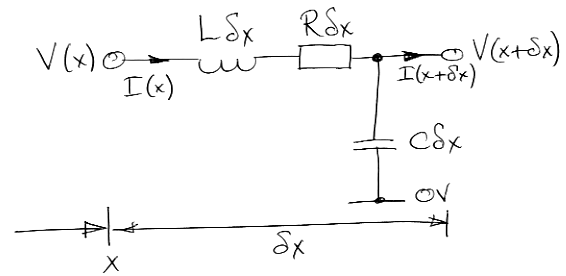Figure: Transmission Line Element. Here we have C, L, and R as the capacitance, inductance, and resistance per unit length of line. Voltage with distance and time is V(x,t), and current is I(x,t).

Our element is a short length, δx, of cable. Distance along the cable is x. Although the capacitance, inductance, and resistance of a transmission line are distributed and mingled with one another, we lump them into three separate components in our infinitesimal element. As δx→0, our lumped model becomes a distributed model. Consider the voltage across the inductor and resistor. At position x and time t a current I(x, t) passes through both of them in series.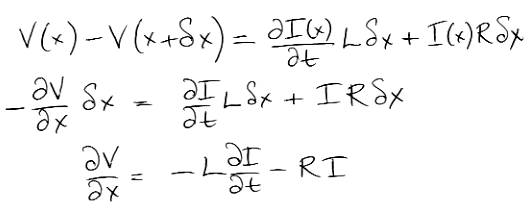Equation 1: Voltage Across Inductive Element.

The rate of change of voltage with x at a particular time is a function of the rate of change of current with time and the current itself.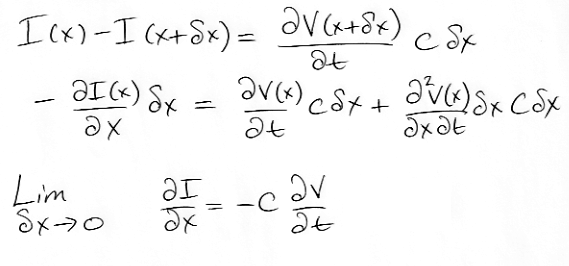Equation 2: Current Into Capacitive Element.

The rate of change of current with x at a particular time is proportional to the rate of change of voltage with time. Let us differentiate (1) with respect to x and (2) with respect to t. We use the resulting equations to eliminate terms in I.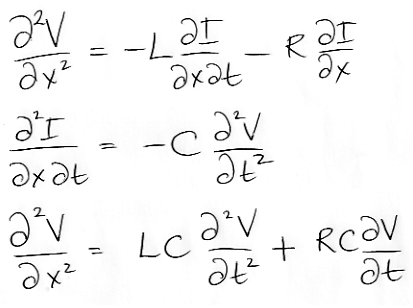Equation 3: Transmission Line Equation.

We arrive at a partial differential equation in V. If we assume R is zero, we are left with the second derivative in x being proportional to the second derivative in t. These are the conditions under which a sinusoidal wave will propagate without distortion or attenuation. Consider a sinusoid of frequency f = ω/2π, as shown below.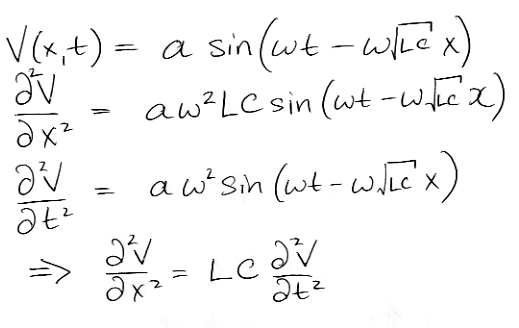Equation 4: Propagating Sine Wave.

The sinusoidal wave has the unique property that its derivatives have the same shape as the original. There is some scaling of the amplitude of the waveform as we differentiate, and it is this scaling that constrains the solution to our transmission line equation. If we set t = √(LC)x, we see the movement of the positive zero-crossing of the sinusoid (the value of sine when its angle is zero). We have dx/dt = 1/√(LC). The velocity of the sine wave is 1/√(LC). Provided that L and C remain constant with ω, the velocity of all sine waves will be the same. Let V(0, t) denote the voltage at position zero and time t. If we represent our input V(0, t) as a sum of sinusoids using a Fourier transform, all these sinusoids will propagate along the transmission line at the same speed, so that their sum will remain undistorted as it propagates, and we will have our ideal transmission line: V(x, t) = V(0, t − τ) with τ = x √(LC).

## Characteristic Impedance

Consider the relationship between voltage and current at the input of our transmission line.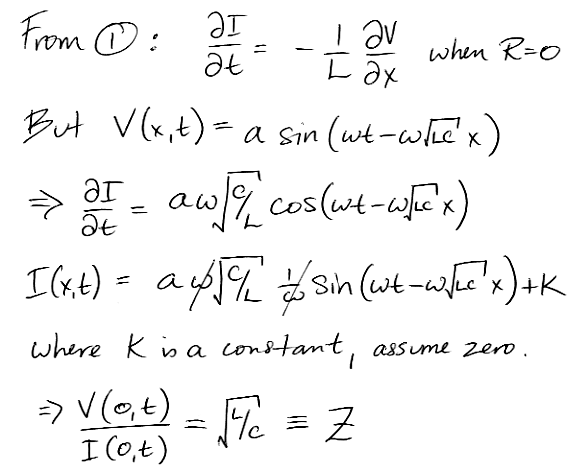Equation 5: Characteristic Impedance of a Transmission Line.

When we let R = 0, we see that V(x)/I(x) is not a function of t, nor even of x. At any x, V/I = √(L/C). So far as the source of V(0, t) is concerned, the transmission line behaves in exactly the same way as a resistor of value √(L/C). We call this resistance the characteristic impedance of the transmission line. The characteristic impedance of free space, for waves propagating through a vacuum, is 377 Ω. The characteristic impedance of water is 42 Ω. Waves propagate through a vacuum at 299 m/μs, but they propagate through water at only 34 m/μs.

## Common Lines

The following table gives the values of L and C for various mediums, including free space. The capacitance of free space is the same as the permittivity of free space. The inductance of free space is the same as the permeability of free space.

Medium C (pF/m) L (nH/m) v (m/μs) Z (Ω) R for f≤ 1kHz
(mΩ/m)
RG58/U Coaxial Cable93.52731985453
RG58C/U Coaxial Cable1012521985050
RG59B/U Coaxial Cable72.04051857545
CAT-5 Twisted Pair (Solid)49.2495203100180
Vacuum8.8512602993770
Water708126034420
Table: Wave Velocity and Characteristic Impedance of Various Mediums. Each medium acts as a transmission line. The velocity is v and the characteristic impedance is Z.

The high-frequency resistance of wires is proportional to √ω, due to the skin effect, which we will discuss later. For now, the table gives R for f = ω/2π ≤ 1 kHz. We obtained R for the cables by adding the conductor and shield DC resistance per meter. In a vacuum, there is no heat-generating resistance to the movement of charges so R = 0. We are not confident that we can defend our assignation of R = 0 in water, but that's our best guess. Also in the table is the characteristic impedance of the medium.

## General Solution

Consider the case when R > 0. We have the second-order differential equation in V given above. Instead of a simple sinusoidal solution, we propose a solution in which the sinusoid amplitude decreases exponentially with distance as it propagates along the cable at a fixed velocity. We have good reason to suggest a solution of this form. No circuit made of capacitors, inductors, and resistors can distort the shape of a sinusoid, so we are confident that a sinusoid propagating down a transmission line will remain a sinusoid. Furthermore, if we consider the case when R is small, the cable beyond an element of transmission line behaves like a pure resistance, Z. The element's resistance will divide the sinusoidal amplitude by 1/(1+Rδx/Z), which implies exponential attenuation with distance.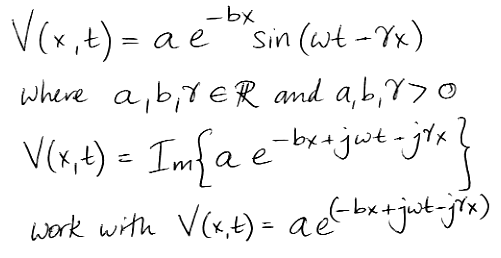Equation 6: General Solution for R > 0. We use j for √−1 and use the complex exponential identity e = cosθ + j sinθ. In our derivations of the general solution, we work with a complex expression for V(t) because doing so greatly abbreviates and clarifies our equations. But our actual applied voltage is the imaginary part of V(t) and the actual currents and voltages farther down the transmission line are the imaginary parts of our complex-valued solutions.

We use γ to denote the wavenumber of the signal propagating down the transmission line. We have γ = 2π/λ, where λ is the wavelength. (The letter k is also popular for denoting the wave number.) The parameter b is the attenuation constant, which defines how the amplitude of our sine wave decreases with distance along the line. In the case where R = 0, we would have b = 0 and γ = ω√(LC). With the complex exponential representation, our derivatives are simpler, as you can see below.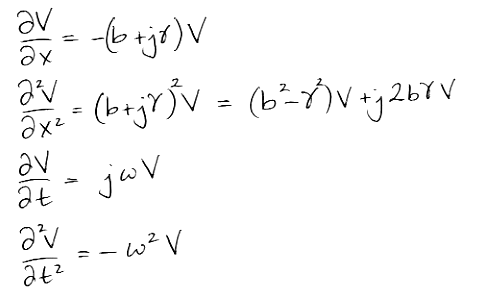Equation 7: Derivatives of the General Solution.

Now we substitute the Derivatives of the General Solution (7) into the Transmission Line Equation (3). We compare the real and imaginary parts of the resulting identity and so obtain two equations in b and γ, which we solve for γ to obtain Equation 8.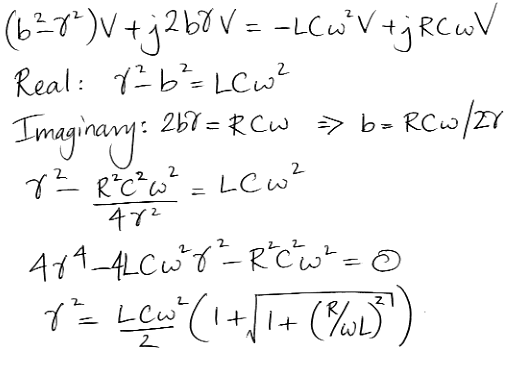Equation 8: Wavenumber of Propagating Sinusoid for R > 0.

The wave velocity, v = ω/γ, is the speed with which a peak in the wave propagates along the transmission line. The wavelength, λ = 2π/γ, is the distance between peaks in the wave at a particular point in time. The following table gives γ, v, and λ under various conditions.

Condition γ v λ
R = 0 ω√(LC) 1 / √(LC) 2π / √(LC)
ωL >> R > 0 ω√(LC) 1 / √(LC) 2π / √(LC)
ω → ∞ ω√(LC) 1 / √(LC) 2π / √(LC)
ωL = R > 0 1.1 ω√(LC) 0.91 / √(LC) 1.8 π / √(LC)
ωL << R 0.71 √(ωRC) 0.71 √(ω / RC) 1.4 π / √(ωRC)
Table: Wavenumber (γ), Wave Velocity (v), and Wavelength (λ) Under Various Conditions.

As we increase ω from zero, the wave velocity increases from zero and approaches 1 / √(LC). High-frequency waves travel more quickly than low-frequency waves. Consider RG58/U cable for which R = 53 mΩ/m. At 1 kHz, we have ωL = 1.7 mΩ/m << R = 53 mΩ/m so v = 25 m/μs, but as ω → ∞ we have v → 200 m/μs. High-frequency waves travel eight times faster down RG58/U cable than low-frequency waves.

Before we start imagining high-frequency waves getting ahead of the low-frequency waves, let us solve for the attenuation constant, b. If the high-frequency waves are attenuated more quickly than low frequency waves, it won't matter how much the high-frequency waves get ahead of the low-frequency waves, because the high-frequency waves will become vanishingly small.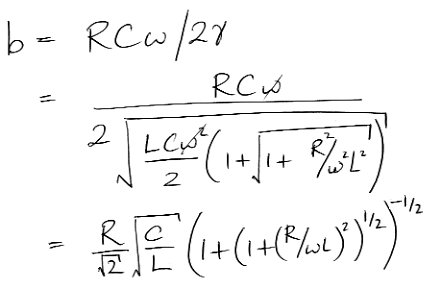Equation 9: Attenuation Constant of Propagating Sinusoid for R > 0.

Here we see two familiar terms in the solution for b. We have √(C/L) ≡ 1/Z and also RL. The wave amplitude after one meter is a exp(−b). The following table gives b and exp(−b) under various conditions.

Condition b (1/m) exp(−b × 1 m)
R = 0 0 1
ωL >> R > 0 0.50 R√(C / L) = 0.50 R / Z exp(−0.50 R/Z)
ω → ∞ 0.50 R√(C / L) = 0.50 R / Z exp(−0.50 R/Z)
ωL = R > 0 0.45 R√(C / L) = 0.45 R / Z exp(−0.45 R/Z)
ωL << R 0.71 √(ωRC) exp(−0.71 √(ωRC))
Table: Attenuation Constant and Relative Amplitude After One Meter Under Various Conditions.

Consider RG58/U cable at 1 kHz. We have Z = 54 Ω, ωL = 1.7 mΩ/m << R = 53 mΩ/m. So b = 0.00012 /m and the attenuation will be 0.001 dB/m. If R did not increase with frequency, but remained constant at 53 mΩ/m, as ω→∞ we would have b→0.00049 /m and the attenuation would be 0.0043 dB/m at high frequencies. Meanwhile, our 1-kHz waves would move at 25 m/μs (see above) and our high-frequency waves would move at 200 m/μs (see above). If R remains constant with frequency, when we apply a step change in V to one end of a 100-m long RG58/U cable, the rising edge of the step will reach the other end in 0.5 μs and the 1 kHz component will arrive in 4 μs, with neither of them being attenuated by more than 5%. As we shall see in the next section, however, the resistance increases with frequency.

## Skin Effect

The skin effect is the restriction of alternating current to the surface of a conductor. This restriction is caused by the alternating magnetic field that the current itself generates within the conductor. The higher the frequency of the alternation, the thinner the layer of conductor into which the current will be driven by these magnetic fields. We derive equations for skin depth, δ, and skin resistance, Rs in The Skin Effect. At sufficiently high frequencies, a cylindrical conductor of radius r will have resistance per unit length given by:

R = √(ρ f μ) / (2 r √π).

The above relation applies to a 400-μm radius copper wire provided that the skin depth is much smaller than the wire radius, which is true for f >> 17 kHz (see The Skin Effect for proof). At high enough frequencies, the resistance of any copper wire of radius r will be:

R = 41 √f / r nΩ/m

For the 400-μm radius copper wire at the center of an RG58/U coaxial cable, the resistance per unit length at f = 1 GHz is R = 3.2 Ω/m. The attenuation constant is b = 0.030 /m (0.5 × R / Z, and Z = 54 Ω for RG58/U). The attenuation of 100 m of the central conductor is 26 dB. The return conductor also presents resistance. It is the coaxial cable shield. The shield radius is 1.8 mm, but it is made of stranded wires, so it is not clear that we can apply the above formula directly. But suppose the shield were another cylinder of radius 1.8 mm, then its resistance would be R = 0.72 Ω/m at 1 GHz and it would add another 6 dB of attenuation. The total attenuation per 100 m would be 32 dB. As we will see in the next section, the actual attenuation of such cable at 1 GHz is closer to 50 dB for 100 m.

## Surface Roughness

When we look up the actual attenuation of RG58/U cable at 1 GHz, we find it to be anywhere from 0.3 dB/m to 0.5 dB/m, depending upon the manufacturer, even though all RG58/U has the same diameter copper conductor and shield. We measured the attenuation of several cables at 900 MHz, as we show here, and our measurements agreed roughly with the manufacturer's data sheets.

We calculated that the attenuation of RG58/U at 1 GHz should be around 0.32 dB/m. The actual attenuation is higher because of surface roughness and impurities in the copper, primarily the former. The skin depth of 1 GHz in copper is only 2 μm. In order to obtain the 0.32 dB/m performance of our prediction, the surface of the copper conductor must be polished to better than 1 μm, or else the skin through which the current flows will be an undulating series of valleys and mountains that greatly increase the effective length of the wire. In order to obtain 0.32 dB/m, the surface of the copper must be optically flat (the wavelength of light is roughly half a micron). Furthermore, any surface corrosion of the copper will dramatically reduce the conductivity of the surface material. Thus our 0.32 dB/m at 1 GHz is the theoretical minimum resistance of a 400-μm radius copper conductor with surface roughness ±200 nm and no corrosion or impurities in the surface copper.

The following table shows the perfect-surface attenuation for RG58/U, accounting for its 400-μm radius central copper conductor and its shield, and compares it to the observed attenuation at range of frequencies.

Frequency (MHz) Perfect (dB/100m) Measured (dB/100m)
11.51.3
103.74.0
507.710
1001014
2001520
5002334
10003355
Table: Comparison of Calculated and Measured Attenuation in RG58/U Cable.

The perfect and measured values are in excellent agreement at 1 MHz, poor agreement at 100 MHz, and gross disagreement at 1 GHz, where the measured attenuation is 22 dB higher than our calculation. The typical surface roughness of drawn copper wire is around 2 μm (0.0001"). At 100 MHz, the skin depth in copper is 6.6 μm, so surface roughness will start to affect the resistance of the wire. At 1 GHz the skin depth is only 2 μm, so the path of the current is dominated by scratches and surface corrosion. To carry a 1-GHz signal more than a few meters with metal conductors, we must polish the conductor surface. High-performance coaxial cables have polished silver conductors in the center.

## Distortion

According to our analysis, higher-frequency signals propagate more quickly down real transmission lines than low-frequency signals. But high-frequency signals are attenuated more quickly. A 100-m length of RG58/U cable will attenuate the amplitude of a 1-GHz signal by 55 dB. A 1-kHz signal, meanwhile, will be attenuated by only 0.10 dB. The 1-GHz signal will get to the end of the cable in 500 ns, but it will be almost six hundred times smaller in amplitude. The 1-kHz signal will get to the end in 4 μs and it will be almost as large as it started. The separation and attenuation of different frequencies by a transmission line causes distortion of the signals it carries. The longer the transmission line, the greater the distortion. The higher the frequency of the input, the greater the distortion. When we apply a step input, we will find that the sharp edge becomes rounded off as it moves farther along the cable.

The greatest source of distortion in transmission lines is the skin effect, which increases the effective resistance of the conductors with frequency. Some authors describe the rounding off of a sharp step along a transmission line as being the result of dispersion. But dispersion is the propagation of different frequencies at different speeds. For practical cables like RG58/U, distortion is dominated by the attenuation of higher frequencies, not by the dispersion of higher frequencies. And in any case: what dispersion there is works to slow down the low-frequency signals, not the high-frequency signals.

For a case study in the failure of digital communication because of a combination of slow low-frequency components and attenuated high-frequency components, see here.

## Reflection Coefficient

Suppose we have a sinusoidal wave propagating down an ideal transmission line with characteristics impedance ZT. The transmission line ends with a load impedance ZL. In the transmission line, the wave's voltage is ZT times its current. But the load voltage must be ZL times the load current. If ZT = ZL, then the wave voltage will appear on the load and all the wave current will flow through the load. But if ZTZL, the current flowing into the load will not match the wave current, and we must ask ourselves how this apparent conflict can be resolved electrically. The answer is that it is possible for another wave to be generated by the load, a wave that propagates in the opposite direction, back to the source of the original wave. We will call the original wave the forward wave, and the returning wave the reflected wave. Because the reflected wave will propagate back up the transmission line, so the ratio of its voltage to current must also be equal to ZT.

The reflection coefficient, which we denote with the symbol Γ, is the ratio of the reflected wave amplitude to the forward wave amplitude. We derive an expression for Γ below, in terms of ZT and ZL. In the derivation below, we assume the existence of a reflected wave. The voltage on the load is the sum of the forward and reflected wave voltages, but the current in the load is the difference between the forward and reflected wave currents.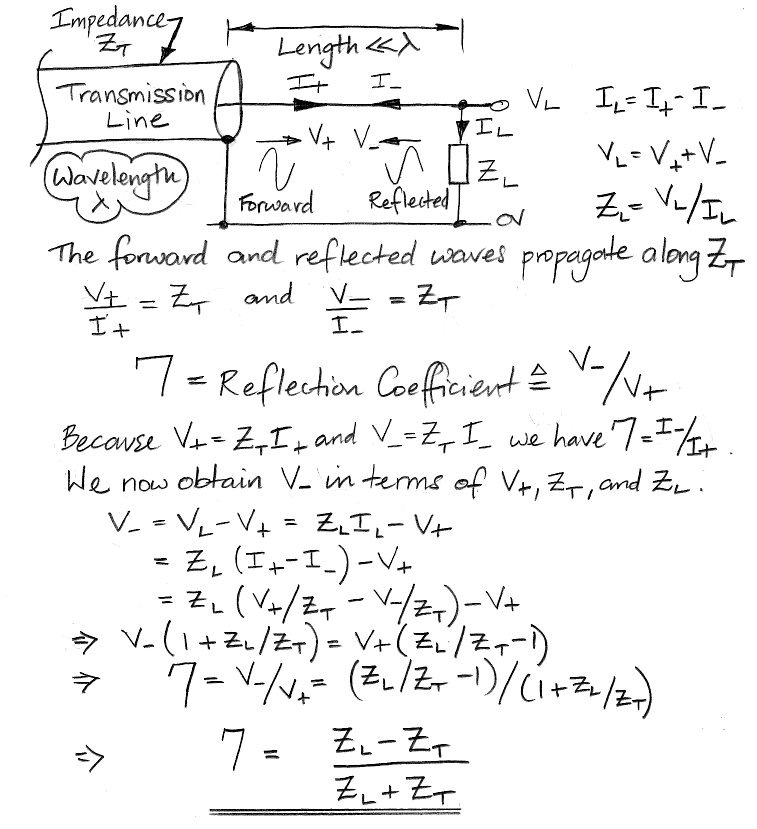Figure: Derivation of Expression for Reflection Coefficient in Terms of Transmission Line and Load Impedance. In our drawing, we show a close-up of the load so that we can draw the currents and voltages in place, and so we note that the exposed length of conductor in our drawing is far shorter than the length of the forward and reflected waves, so that no change in either wave takes place before it meets the load.

When ZL = ZT we have Γ = 0, and there is no reflection.

The forward wave carries energy. The voltage source that applied the wave to the far end of the transmission line delivered electrical energy to ZT. The distributed inductance and capacitance of the transmission line absorb energy temporarily, but surrender it again as the wave propagates. The ideal transmission line does not dissipate the energy. It merely transports the energy. When the energy arrives at ZL, it either enters the load or it reflects back to the source. If ZL is entirely capacitive or inductive, the load will not absorb any energy either. In that case, the magnitude of the reflection coefficient must be one, because all the wave energy will have to travel back to the source. The same argument applies to the case when ZL is 0 Ω (short circuit) or ∞ Ω (open circuit). The following calculation confirms that Γ does indeed conform to the law of conservation of energy.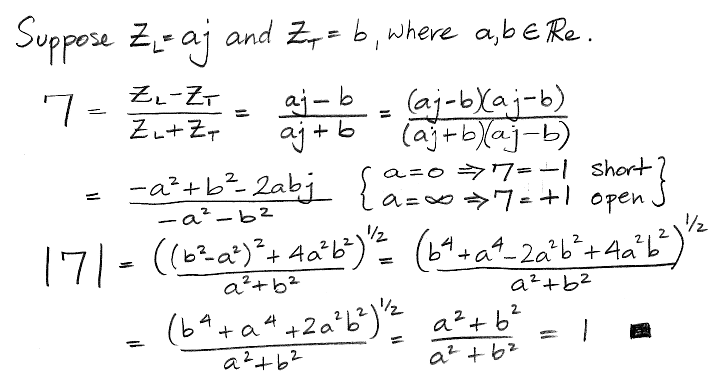Figure: Reflection Coefficient for Loads that Do Not Absorb Energy.

We see that the short circuit termination, where ZL = 0, results in Γ = −1. The entire forward wave is reflected with its voltage and current negated. Because the voltage of the reflected wave is negated, the net voltage on the load is zero. Because the current is negated, and it was in the first place assumed to be going in the opposite direction to that of the forward wave, the current flowing into the load is twice the current of the forward wave. For ZL = ∞ the entire forward wave is reflected with its voltage intact. The voltage on the load is double the forward wave's voltage, and the current into the load is zero.

## Transmission Line Stubs

When a forward wave reflects off the load at the far end of a transmission line, the reflected wave returns to the near end. When it arrives at the near end, it adds to the forward wave, and so alters the ratio of voltage to current at the near end. Thus the reflection alters the impedance of the transmission line, as seen by any voltage source connected to the near end. The value of the load impedance, ZL, determines the reflection coefficient, while the length, L, of the transmission line determines the phase of the reflected wave at the near end. It turns out that we can create any impedance we like at the near end by our choice of ZL and L. We call such a line a transmission line stub.

We can use a stub to introduce an impedance into a circuit instead of using capacitors, inductors, and resistors. Stubs with open-circuit and short-circuit terminations are often used in radio-frequency matching networks. In the figure below, we derive the impedance at the near end in terms of L, ZL, and the characteristics impedance of the line, ZT. In our derivation, we represent voltages, currents, and phase shifts with complex exponentials.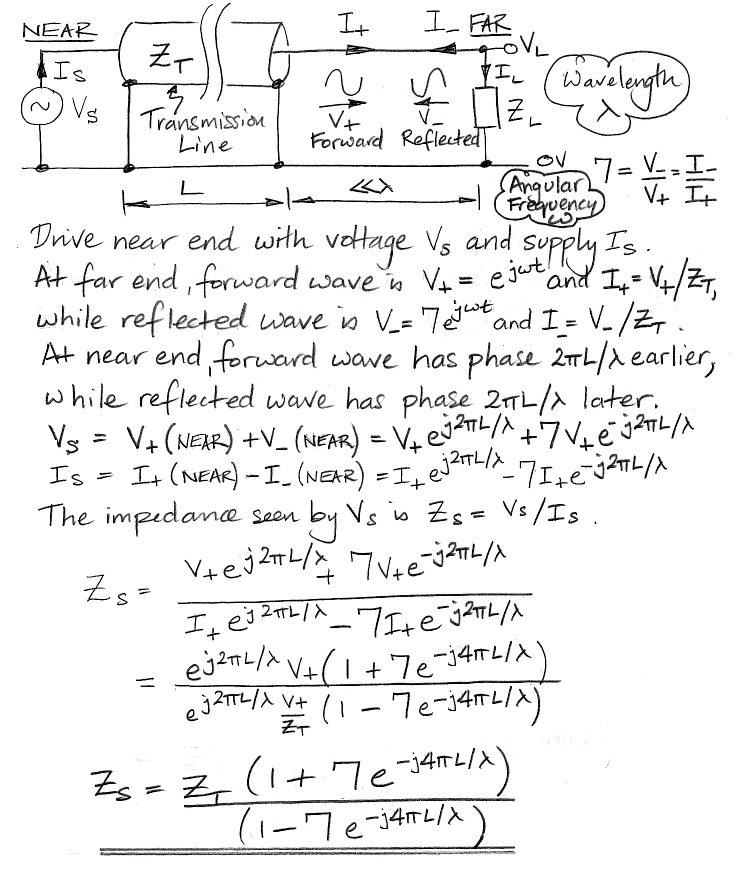Figure: Transmission Line Stub Impedance.

The terms e−j4πL/λ represent the phase delay of a wave traveling from the near to the far end and back again. The numerator represents the sum of the forward and reflected voltages at the near end, while the denominator represents the difference between the forward and reflected currents at the near end. The following table gives some example stub impedances for various stub lengths and load impedances.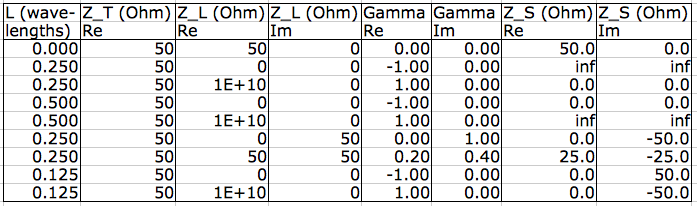Table: Example Stub Impedances. The stub length, L, is in units of wavelength. The characteristic impedance, ZT, we assume is resistive. The load impedance, ZL, has a real and imaginary part. We calculate the real and imaginary parts of the reflection coefficient, Gamma. We calculate the real and imaginary parts of the reflection coefficient (R.C.). We obtained our table with our stub impedance calculation spreadsheet, available here. We use "1e10" as an open-circuit load. And "inf" result means an open circuit stub impedance.

A quarter-wavelength stub with an open-circuit load has impedance 0 Ω, while the same stub with a short-circuit load has infinite impedance. A one-eighth wavelength stub with a short-circuit load produces an inductive impedance of the same magnitude as the transmission line impedance (50j Ω for a 50-Ω transmission line). An open-circuit load on the same stub produces a capacitive impedance (−50j Ω).

## Conclusion

Our discusion answers the most important questions about the behavior of transmission lines. We show that it is the attenuation of higher frequencies by the skin effect that causes the degradation of voltage transitions on long transmission lines, not the dispersion of higher frequencies. Indeed, the dispersion alone would cause the opposite effect: higher frequencies propagate far more quickly than lower frequencies. A 1-GHz sinusoid, for example, propagates down a 100-m RG58/U cable in 500 ns, while a 1-kHz sinusoid takes 4 μs. The amplitude of the 1-GHz signal, however, will be attenuated by a factor of six hundred by the time it gets to the other end, while the 1-kHz signal will arrive at almost the same amplitude as it entered. The late-arrival of the low-frequency component of a signal gives rise to settling times of order microseconds at the far end of hundred-meter cables. The attenuation of higher frequencies is dominated by the skin effect, which serves to increase the effective resistance of the transmission line in proportion to the square root of the frequency. Once we get above 100 MHz, the surface polish of the conductor begins to limit the performance of a cable, which explains why the best high-frequency coaxial cables use polished silver conductors.

Because an imperfectly-terminated transmission line causes power to be reflected back to the source, the impedance seen looking into such a line is not equal to the characteristic impedance of the line, but some function of the reflection coefficient at the far end, and the length of the line. If we are working at one particular frequency, or a narrow range of frequencies, we can use a finite transmission line, or stub to create any impedance we like. We can use such stubs as matching networks.